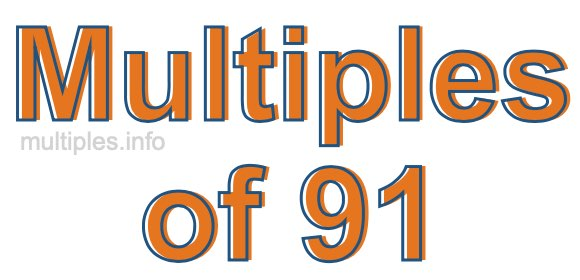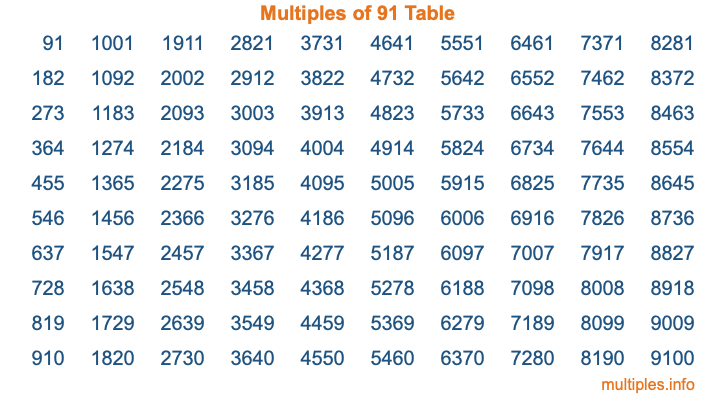Multiples of 91Welcome to the Multiples of 91 page. Here we will first teach you everything you will ever need to know about the multiples of 91, and then give you a study guide summary of everything we taught you to make sure you remember it all. Use this page to look up facts and learn information about the multiples of 91. This page will make you a multiples of ninety-one expert!

Definition of Multiples of 91
Multiples of 91 are all the numbers that when divided by 91 equal an integer. Each of the multiples of 91 are called a multiple. A multiple of 91 is created by multiplying 91 by an integer.

Therefore, to create a list of multiples of 91, you start with 1 multiplied by 91, then 2 multiplied by 91, then 3 multiplied by 91, and so on for as long as you want. Thus, the list of the first five multiples of 91 is 91, 182, 273, 364, and 455. To see a larger list of multiples of 91, see the printable image of Multiples of 91 further down on this page. We also have a category where you can choose any nth multiple of 91.

Multiples of 91 Checker
The Multiples of 91 Checker below checks to see if any number of your choice is a multiple of 91. In other words, it checks to see if there is any number (integer) that when multiplied by 91 will equal your number. To do that, we divide your number by 91. If the the quotient is an integer, then your number is a multiple of 91.

Is  a multiple of 91?

Least Common Multiple of 91 and ...
A Least Common Multiple (LCM) is the lowest multiple that two or more numbers have in common. This is also called the smallest common multiple or lowest common multiple and is useful to know when you are adding our subtracting fractions. Enter one or more numbers below (91 is already entered) to find the LCM.

Check out our LCM Calculator if you need more details about the Least Common Multiple or if you need the LCM for different numbers for adding and subtraction fractions.

nth Multiple of 91
As we stated above, 91 is the first multiple of 91, 182 is the second multiple of 91, 273 is the third multiple of 91, and so on. Enter a number below to find the nth multiple of 91.

th multiple of 91

Multiples of 91 vs Factors of 91
91 is a multiple of 91 and a factor of 91, but that is where the similarities end. All postive multiples of 91 are 91 or greater than 91. All positive factors of 91 are 91 or less than 91.

Below is the beginning list of multiples of 91 and the factors of 91 so you can compare:

Multiples of 91: 91, 182, 273, 364, 455, etc.

Factors of 91: 1, 7, 13, 91

As you can see, the multiples of 91 are all the numbers that you can divide by 91 to get a whole number. The factors of 91, on the other hand, are all the whole numbers that you can multiply by another whole number to get 91.

It's also interesting to note that if a number (x) is a factor of 91, then 91 will also be a multiple of that number (x).

Multiples of 91 vs Divisors of 91
The divisors of 91 are all the integers that 91 can be divided by evenly. Below is a list of the divisors of 91.

Divisors of 91: 1, 7, 13, 91

The interesting thing to note here is that if you take any multiple of 91 and divide it by a divisor of 91, you will see that the quotient is an integer.

Multiples of 91 Table
Below is an image of the first 100 multiples of 91 in a table. The table is in chronological order, column by column. The first column has the first ten multiples of 91, the second column has the next ten multiples of 91, and so on.The Multiples of 91 Table is also referred to as the 91 Times Table or Times Table of 91. You are welcome to print out our table for your studies.

Negative Multiples of 91
Although not often discussed or needed in math, it is worth mentioning that you can make a list of negative multiples of 91 by multiplying 91 by -1, then by -2, then by -3, and so on, to get the following list of negative multiples of 91:

-91, -182, -273, -364, -455, etc.

Multiples of 91 Summary
Below is a summary of important Multiples of 91 facts that we have discussed on this page. To retain the knowledge on this page, we recommend that you read through the summary and explain to yourself or a study partner why they hold true.

There are an infinite number of multiples of 91.

A multiple of 91 divided by 91 will equal a whole number.

91 divided by a factor of 91 equals a divisor of 91.

The nth multiple of 91 is n times 91.

The largest factor of 91 is equal to the first positive multiple of 91.

91 is a multiple of every factor of 91.

91 is a multiple of 91.

A multiple of 91 divided by a divisor of 91 equals an integer.

91 divided by a divisor of 91 equals a factor of 91.

Any integer times 91 will equal a multiple of 91.

Multiples of a Number
Here you can get the multiples of another number, all with the same attention to detail as we did for multiples of 91 on this page.

Multiples of
Multiples of 92
Did you find our page about multiples of ninety-one educational? Do you want more knowledge? Check out the multiples of the next number on our list!

Copyright  |   Privacy Policy  |   Disclaimer  |   Contact